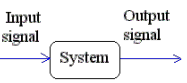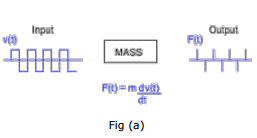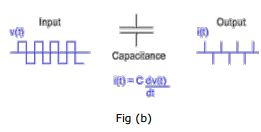# Introduction to Signal & Systems Electrical Engineering (EE) Notes | EduRev

## Electrical Engineering (EE) : Introduction to Signal & Systems Electrical Engineering (EE) Notes | EduRev

The document Introduction to Signal & Systems Electrical Engineering (EE) Notes | EduRev is a part of the Electrical Engineering (EE) Course Signal & Systems.
All you need of Electrical Engineering (EE) at this link: Electrical Engineering (EE)

Introduction

The intent of this introduction is to give the reader an idea about Signals and Systems as a field of study and its applications. But we must first, at least vaguely define what signals and systems are.

• Signals are functions of one or more variables .
• Systems respond to an input signal by producing an output signal .Examples of signals include :
1. A voltage signal: voltage across two points varying as a function of time.
2. A force pattern: force varying as a function of 2-dimensional space.
3. A photograph: color and intensity as a function of 2-dimensional space.
4. A video signal: color and intensity as a function of 2-dimensional space and time

Examples of systems include :

1. An oscilloscope: takes in a voltage signal, outputs a 2-dimensional image characteristic of the voltage signal.
2. A computer monitor: inputs voltage pulses from the CPU and outputs a time varying display.
3. An accelerating mass : force as a function of time may be looked at as the input signal, and velocity as a function of time as the output signal.
4. A capacitance: terminal voltage signal may be looked at as the input, current signal as the output. Examples of mechanical and electrical systems

Examples of mechanical and electrical systems

You are surely familiar with many of these signals and systems and have probably analyzed them as well, but in isolation . For instance, you must have studied accelerating masses in a mechanics course (see Fig (a)), and capacitances in an electrostatic course (see Fig (b)), separately.We will develop very general tools and techniques of analyzing systems, independent of the actual context of their use. Our approach in this course would be to define certain properties of signals and systems (inspired of course by properties real-life examples we have), and then link these properties to consequences. These "links" can then be used directly in connection with a large variety of systems: electrical, mechanical, chemical, biological… knowing only how the input and output signal are related! Thus, our focus when dealing with signals and systems will be on the relationship between the input and output signal and not really on the internals of the system.

Issues that will concern us in signals and systems include
1. Characterization (description of behavior) of systems and signals.
2. Design of systems with certain desired properties.
3. Modification of existing systems to our advantage.

With this introduction, let us go on to formally defining signals and systems.

Conclusion:

In this lecture you have learnt:

Signals are functions of one or more independent variables.
Systems are physical models which gives out an output signal in response to an input signals.
Trying to identify real-life examples as models of signals and systems, would help us in understanding the subject better.

Offer running on EduRev: Apply code STAYHOME200 to get INR 200 off on our premium plan EduRev Infinity!

## Signal & Systems

32 videos|42 docs|9 tests

,

,

,

,

,

,

,

,

,

,

,

,

,

,

,

,

,

,

,

,

,

;